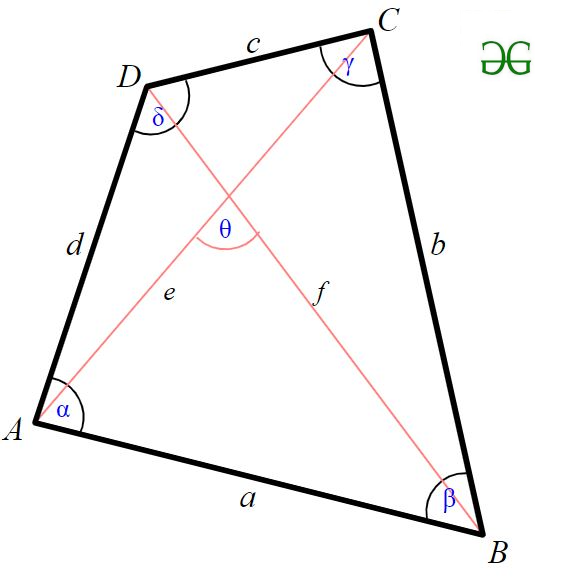Open In App
Related Articles

Given four sides of quadrilateral a, b, c, d, find the maximum area of the quadrilateral possible from the given sides .
Examples:

Input : 1 2 1 2
Output : 2.00
It is optimal to construct a rectangle for maximum area .According to Bretschneider’s formula, the area of a general quadrilateral is given byHere a, b, c, d are the sides of a quadrilateral, s is the semiperimeter of a quadrilateral and angles are two opposite angles.
So, this formula is maximized only when opposite angles sum to pi(180) then we can use a simplified form of Bretschneider’s formula to get the (maximum) area K.This formula is called as Brahmagupta’s formula
Below is the implementation of given approach

## C++

 // CPP program to find maximum area of a// quadrilateral#include #include using namespace std;  double maxArea(double a, double b,                double c, double d){    // Calculating the semi-perimeter    // of the given quadrilateral    double semiperimeter = (a + b + c + d) / 2;      // Applying Brahmagupta's formula to    // get maximum area of quadrilateral    return sqrt((semiperimeter - a) *                (semiperimeter - b) *                (semiperimeter - c) *                (semiperimeter - d));}  // Driver codeint main(){    double a = 1, b = 2, c= 1, d = 2;   cout <

## C

 // CPP program to find maximum area of a// quadrilateral#include #include   double maxArea(double a, double b,                double c, double d){    // Calculating the semi-perimeter    // of the given quadrilateral    double semiperimeter = (a + b + c + d) / 2;      // Applying Brahmagupta's formula to    // get maximum area of quadrilateral    return sqrt((semiperimeter - a) *                (semiperimeter - b) *                (semiperimeter - c) *                (semiperimeter - d));}  // Driver codeint main(){    double a = 1, b = 2, c= 1, d = 2;    printf("%.2f\n",maxArea(a, b, c, d));    return 0;}

## Java

 // Java program to find maximum area of a// quadrilateralimport java.io.*; class GFG{    static double maxArea(double a, double b,                           double c, double d)    {        // Calculating the semi-perimeter        // of the given quadrilateral        double semiperimeter = (a + b + c + d) / 2;             // Applying Brahmagupta's formula to        // get maximum area of quadrilateral        return Math.sqrt((semiperimeter - a) *                         (semiperimeter - b) *                         (semiperimeter - c) *                         (semiperimeter - d));    }         // Driver code    public static void main (String[] args)    {        double a = 1, b = 2, c= 1, d = 2;        System.out.println(maxArea(a, b, c, d));    }} // This code is contributed by sunnysingh

## Python3

 # Python3 program to find maximum# area of a quadrilateralimport math def maxArea (a , b , c , d ):     # Calculating the semi-perimeter    # of the given quadrilateral    semiperimeter = (a + b + c + d) / 2         # Applying Brahmagupta's formula to    # get maximum area of quadrilateral    return math.sqrt((semiperimeter - a) *                    (semiperimeter - b) *                    (semiperimeter - c) *                    (semiperimeter - d)) # Driver codea = 1b = 2c = 1d = 2print("%.2f"%maxArea(a, b, c, d)) # This code is contributed by "Sharad_Bhardwaj".

## C#

 // C# program to find maximum area of a// quadrilateralusing System; class GFG {         static double maxArea(double a, double b,                          double c, double d)    {                 // Calculating the semi-perimeter        // of the given quadrilateral        double semiperimeter = (a + b + c + d) / 2;             // Applying Brahmagupta's formula to        // get maximum area of quadrilateral        return Math.Sqrt((semiperimeter - a) *                         (semiperimeter - b) *                         (semiperimeter - c) *                         (semiperimeter - d));    }         // Driver code    public static void Main ()    {        double a = 1, b = 2, c= 1, d = 2;                 Console.WriteLine(maxArea(a, b, c, d));    }} // This code is contributed by vt_m.

## PHP

 

## Javascript

 

Output:

2.00`

Time Complexity: O(logn)
Auxiliary Space: O(1)

Please suggest if someone has a better solution which is more efficient in terms of space and time.